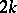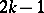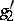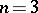Hyper-elliptic curve

(diff) ← Older revision | Latest revision (diff) | Newer revision → (diff)
A non-singular projective model of the affine curve, whereis a polynomial without multiple roots of odd degree(the case of even degreemay be reduced to that of odd degree). The field of functions on a hyper-elliptic curve (a field of hyper-elliptic functions) is a quadratic extension of the field of rational functions; in this sense it is the simplest field of algebraic functions except for the field of rational functions. Hyper-elliptic curves are distinguished by the condition of the existence of a one-dimensional linear seriesof divisors of degree 2, defining a morphism of order 2 of the hyper-elliptic curve onto the projective straight line. The genus of a hyper-elliptic curve is, so that, for various odd, hyper-elliptic curves are birationally inequivalent. Forone obtains the projective straight line; foran elliptic curve is obtained. Traditionally, curves of genus 0 and 1 are not called hyper-elliptic curves. The fractions of regular differential forms generate a subfield of genus 0 on a hyper-elliptic curve of genus; this property is a complete characterization of hyper-elliptic curves.